ID 772987
Date 12/04/2020
Public

## Inverse Transformation

The probability distribution of a one-dimensional variate X may be most generally presented in terms of cumulative distribution function (CDF):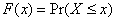.

Any CDF is defined on the whole real axis and is monotonically increasing, where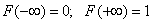.

In case of continuous distribution, the cumulative distribution function F(x) is a continuous one. In what follows, we assume that F(x) is steadily increasing, though assuming a non-steadily increasing function with a limited number of intervals where it steadily increases leads to trivial complications and generalizations of what follows.

Assuming the CDF steadily increases, the following single-valued inverse function should exist: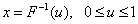It is easy to prove that, if U is a variate with a uniform distribution on the interval (0, 1), then the variate X is of F(x) distribution:Thus, the inverse transformation method can be implemented as follows:

1. Generate a uniformly distributed random number meeting the requirements: 0 < u < 1.

2. Assume x = G(u) as a random number of the distribution F(x).

The only drawback of this approach is that G(u) in a closed form is often hard to find, while numerical solution to the following equation is excessively time-consuming: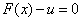For discrete distributions, the CDF is a step function, the inverse transformation method still being applicable. For simplicity, assume that the distribution has probability mass points k = 0, 1, 2, ... with pk probability. Then the distribution function is the sum:,

whereis the maximum integer that does not exceed x. If a continuous function G(u) exists in a closed form so that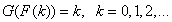,

and G(u) is monotone, then generation of random numbers of the distribution F(x) can be implemented as follows:

1. Generate a uniformly distributed random number meeting the requirements: 0 < u < 1.

2. Assume k = floor(G(u)) as a random number of the distribution F(x).

For example, for the geometric distribution:Then G(u) does exist, as it is easy to prove:However, for most cases finding the closed form for G(u) function is too hard. An acceptable solution may be found using numerical search for k proceeding fromWith tabulated values of F(k), the task is reduced to table lookup. As F(k) is a monotonically increasing function, you may use search algorithms that are considerably more efficient than exhaustive search. The efficiency is solely dependent on the size of the table.

You can apply an inverse transformation method to the s-dimensional quasi-random vectors. The resulting quasi-random sequence has the required s-dimensional non-uniform distribution.